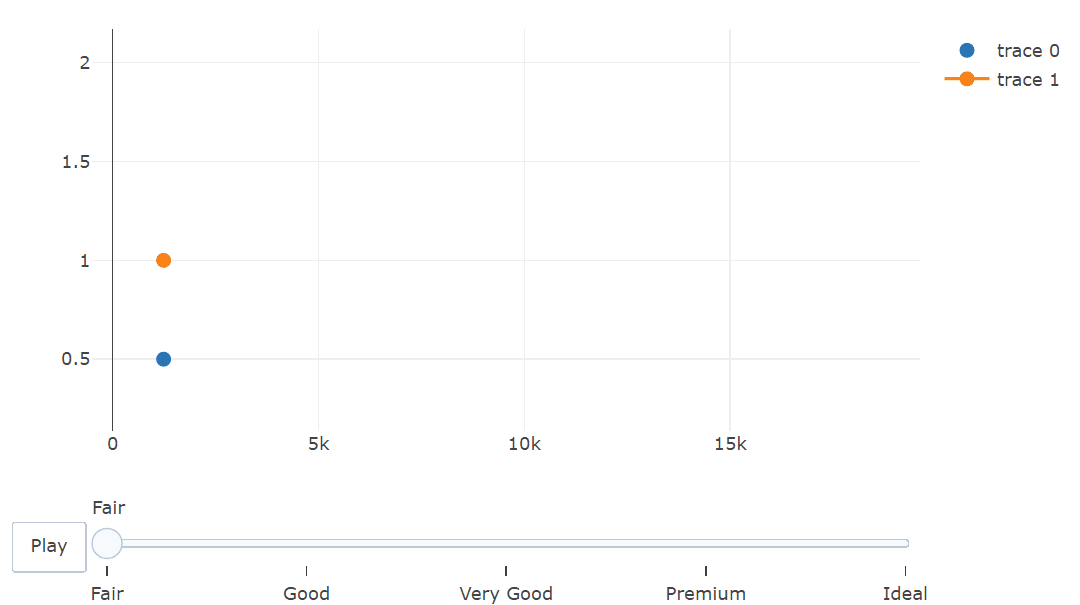Open in App
Not now

# How to Add Constant Line to Animated Plot in Plotly?

• Last Updated : 23 Jan, 2023

Animated plots are a useful way to visualize data over time or to highlight the relationship between different variables. In this article, we will learn how to create animated plots using the plot_ly() function in R Programming Language.

To create an animated plot in R, we will use the plot_ly() function from the plotly package. This function allows us to create a variety of interactive plots, including line plots, scatter plots, and bar plots. We can also use the add_lines() function to add constant lines to the plot.

Install and load the necessary packages: Plotly and ggplot2.

## R

 `install.packages``(``"plotly"``)``install.packages``(``"ggplot2"``)`` ` `library``(plotly)``library``(ggplot2)`

Load the data that you want to use for the plot.

## R

 `data <- ``read.csv``(``"data.csv"``)`

Create the plot using the plot_ly() function. Specify the variables to use for the x-axis, y-axis, and frame (for the animation). Set the type argument to “scatter”, “bar”, or “line” and the mode argument to “lines”, “markers”, or “text”.

## R

 `p <- ``plot_ly``(``  ``x = data\$x,``  ``y = data\$y,``  ``frame = data\$time,``  ``type = ``"scatter"``,``  ``mode = ``"lines"``)`

Use the add_lines() function to add constant lines to the plot. Specify the value for the constant line in the y argument.

## R

 `p <- ``add_lines``(p, y = ``rep``(1, ``length``(data\$x)))`

Example: This will create an animated scatter plot with points colored according to the value of the cut variable in the diamonds dataset. The plot will also include a constant line at y = 1.

## R

 `library``(plotly)``library``(ggplot2)``library``(dplyr)`` ` `# Select a small sample of the data``diamonds_sample <- ``sample_n``(diamonds, size = 50)`` ` `# Create the plot``p <- ``plot_ly``(``  ``x = diamonds_sample\$price,``  ``y = diamonds_sample\$carat,``  ``type = ``"scatter"``,``  ``mode = ``"markers"``,``  ``frame = diamonds_sample\$cut,``  ``marker = ``list``(size = 10)``)`` ` `# Add a constant line at y = 1``p <- ``add_lines``(p,``               ``y = ``rep``(1, ``length``(diamonds_sample\$price)))`` ` `p`

Output:Constant Line to Animated Plot in Plotly

My Personal Notes arrow_drop_up